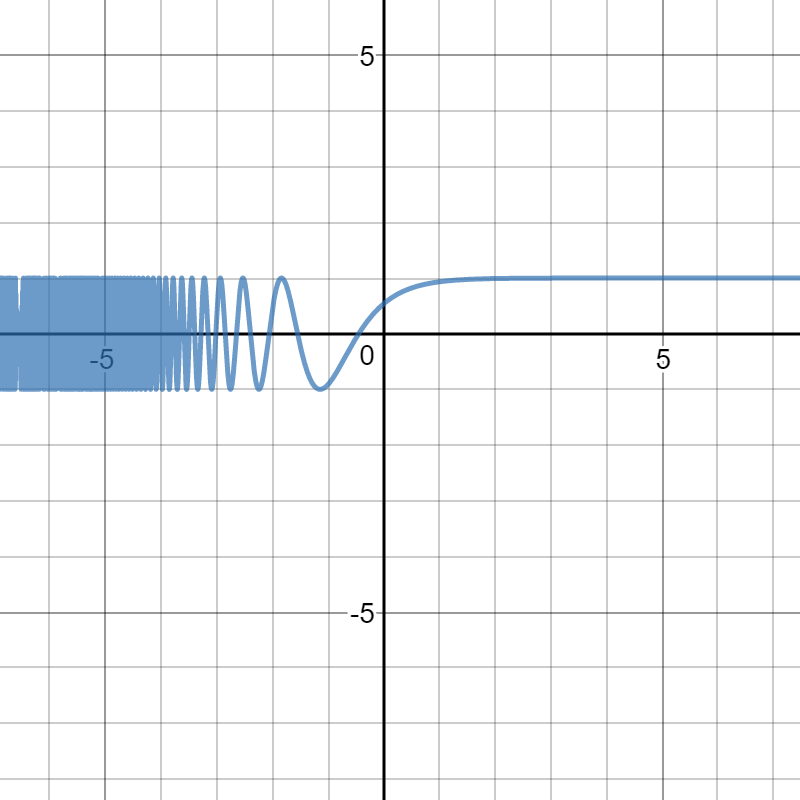# Nature of $y=\cos(e^{-x})$Can anyone explain me the nature of the graph of $y=\cos(e^{-x})$?

You can tell me why this graph behaves in such a way, how to calculate it's period, why the period is becoming infinite when $x$ approaches $-\infty$, etc.

In other words, tell me everything you can know about the properties of the graph.Note by Vilakshan Gupta
2 years, 8 months ago

This discussion board is a place to discuss our Daily Challenges and the math and science related to those challenges. Explanations are more than just a solution — they should explain the steps and thinking strategies that you used to obtain the solution. Comments should further the discussion of math and science.

When posting on Brilliant:

• Use the emojis to react to an explanation, whether you're congratulating a job well done , or just really confused .
• Ask specific questions about the challenge or the steps in somebody's explanation. Well-posed questions can add a lot to the discussion, but posting "I don't understand!" doesn't help anyone.
• Try to contribute something new to the discussion, whether it is an extension, generalization or other idea related to the challenge.

MarkdownAppears as
*italics* or _italics_ italics
**bold** or __bold__ bold
- bulleted- list
• bulleted
• list
1. numbered2. list
1. numbered
2. list
Note: you must add a full line of space before and after lists for them to show up correctly
paragraph 1paragraph 2

paragraph 1

paragraph 2

[example link](https://brilliant.org)example link
> This is a quote
This is a quote
    # I indented these lines
# 4 spaces, and now they show
# up as a code block.

print "hello world"
# I indented these lines
# 4 spaces, and now they show
# up as a code block.

print "hello world"
MathAppears as
Remember to wrap math in $$ ... $$ or $ ... $ to ensure proper formatting.
2 \times 3 $2 \times 3$
2^{34} $2^{34}$
a_{i-1} $a_{i-1}$
\frac{2}{3} $\frac{2}{3}$
\sqrt{2} $\sqrt{2}$
\sum_{i=1}^3 $\sum_{i=1}^3$
\sin \theta $\sin \theta$
\boxed{123} $\boxed{123}$

Sort by:

- 2 years, 8 months ago

It's obvious that there's no period.

- 2 years, 8 months ago

Okay, there's no period, but can you tell me why is the graph becoming compact, when $x$ becomes more negative?

- 2 years, 8 months ago

I want that, if we are not given the graph , how and what conditions we need to find to draw the graph by hand?

- 2 years, 8 months ago

- 2 years, 8 months ago

Thank you.

- 2 years, 8 months ago